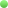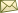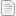Registered: Groups: Anyone, RegisteredSend Email to ADSW1243
 Date Subject Count LocationRe: Vector Poisson Equation for a 3D Current Source 0 replies Modeling and SimulationRe: Vector Poisson Equation for a 3D Current Source 2 replies Modeling and SimulationRe: 3D Magnetostatics in the presence of a current source. 2 replies Basic UseRe: Vector Poisson Equation for a 3D Current Source 0 replies Modeling and SimulationRe: 3D Magnetostatics in the presence of a current source. 6 replies Basic UseRe: 3D Magnetostatics in the presence of a current source. 8 replies Basic UseRe: 3D Magnetostatics in the presence of a current source. 10 replies Basic UseRe: Vector Poisson Equation for a 3D Current Source 5 replies Modeling and SimulationVector Poisson Equation for a 3D Current Source 7 replies Modeling and SimulationRe: 3D Magnetostatics in the presence of a current source. 12 replies Basic UseRe: 3D Magnetostatics in the presence of a current source. 0 replies Basic UseRe: 3D Magnetostatics in the presence of a current source. 15 replies Basic UseRe: 3D Magnetostatics in the presence of a current source. 17 replies Basic UseRe: 3D Magnetostatics in the presence of a current source. 19 replies Basic UseRe: 3D Magnetostatics in the presence of a current source. 0 replies Basic UseImporting gmsh mesh file with several subdomains 22 replies Basic Use